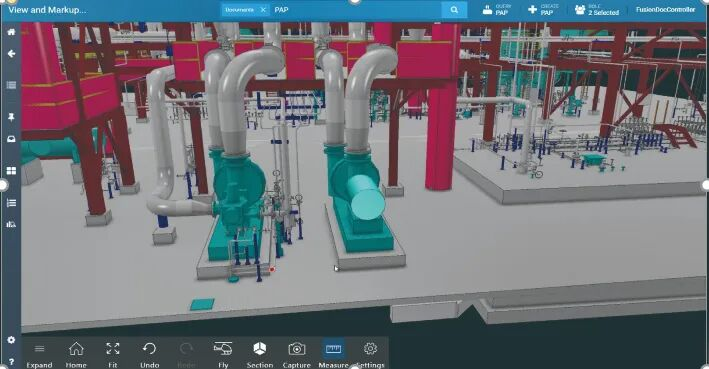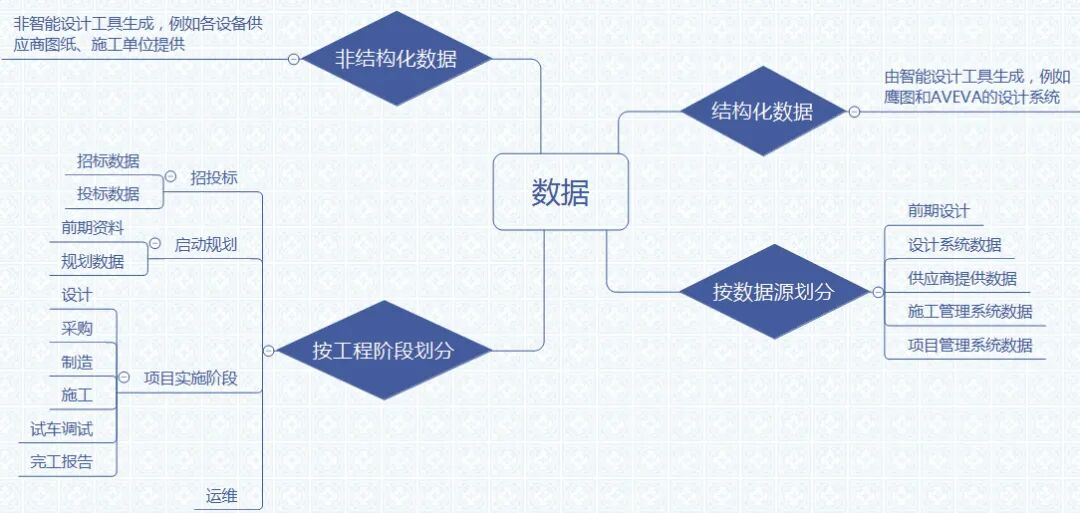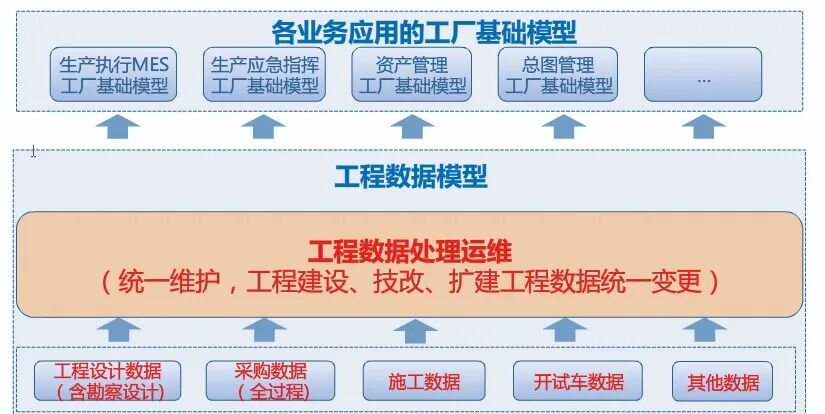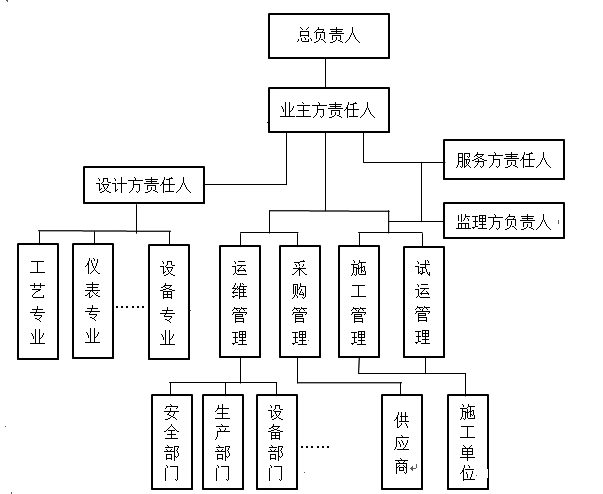400-868-1122• 后市场服务
• 能耗管理
• 设备资产管理
• 融资租赁
• 云应用
• 工业可视化
• AI&大数据分析
• 云物联
• 姓名*

• 公司名称*

• 手机*

• 北京
• 天津
• 河北
• 山西
• 内蒙古
• 辽宁
• 吉林
• 黑龙江
• 上海
• 江苏
• 浙江
• 安徽
• 福建
• 江西
• 山东
• 河南
• 湖北
• 湖南
• 广东
• 广西
• 海南
• 重庆
• 四川
• 贵州
• 云南
• 西藏
• 陕西
• 甘肃
• 青海
• 宁夏
• 新疆
• 香港
• 澳门
• 台湾

• 东城区
• 西城区
• 崇文区
• 宣武区
• 朝阳区
• 丰台区
• 石景山区
• 海淀区
• 门头沟区
• 房山区
• 通州区
• 顺义区
• 昌平区
• 大兴区
• 怀柔区
• 平谷区
• 密云县
• 延庆县
• 和平区
• 河东区
• 河西区
• 南开区
• 河北区
• 红桥区
• 塘沽区
• 汉沽区
• 大港区
• 东丽区
• 西青区
• 津南区
• 北辰区
• 武清区
• 宝坻区
• 宁河县
• 静海县
• 蓟　县
• 石家庄市
• 唐 山 市
• 秦皇岛市
• 邯 郸 市
• 邢 台 市
• 张家口市
• 承 德 市
• 沧 州 市
• 廊 坊 市
• 衡 水 市
• 太原市
• 大同市
• 阳泉市
• 长治市
• 晋城市
• 朔州市
• 晋中市
• 运城市
• 忻州市
• 临汾市
• 吕梁市
• 呼和浩特市
• 包头市
• 乌海市
• 赤峰市
• 通辽市
• 鄂尔多斯市
• 呼伦贝尔市
• 巴彦淖尔市
• 乌兰察布市
• 兴安盟
• 锡林郭勒盟
• 阿拉善盟
• 梅州市
• 中山市
• 韶关市
• 汕头市
• 深圳市
• 珠海市
• 广州市
• 沈阳市
• 大连市
• 鞍山市
• 抚顺市
• 本溪市
• 丹东市
• 锦州市
• 营口市
• 阜新市
• 辽阳市
• 盘锦市
• 铁岭市
• 朝阳市
• 葫芦岛市
• 长春市
• 吉林市
• 四平市
• 辽源市
• 通化市
• 白山市
• 松原市
• 白城市
• 延边朝鲜族自治州
• 哈尔滨市
• 齐齐哈尔市
• 鸡西市
• 鹤岗市
• 双鸭山市
• 大庆市
• 伊春市
• 佳木斯市
• 七台河市
• 牡丹江市
• 黑河市
• 绥化市
• 大兴安岭地区
• 黄浦区
• 卢湾区
• 徐汇区
• 长宁区
• 静安区
• 普陀区
• 闸北区
• 虹口区
• 杨浦区
• 宝山区
• 闵行区
• 嘉定区
• 浦东新区
• 金山区
• 松江区
• 青浦区
• 南汇区
• 奉贤区
• 崇明县
• 南京市
• 无锡市
• 徐州市
• 常州市
• 南通市
• 连云港市
• 淮安市
• 盐城市
• 扬州市
• 镇江市
• 泰州市
• 宿迁市
• 杭州市
• 宁波市
• 温州市
• 嘉兴市
• 湖州市
• 绍兴市
• 金华市
• 衢州市
• 舟山市
• 台州市
• 丽水市
• 合肥市
• 淮南市
• 马鞍山市
• 淮北市
• 铜陵市
• 安庆市
• 黄山市
• 滁州市
• 阜阳市
• 宿州市
• 六安市
• 亳州市
• 池州市
• 宣城市
• 福州市
• 厦门市
• 莆田市
• 三明市
• 泉州市
• 漳州市
• 南平市
• 龙岩市
• 宁德市
• 南昌市
• 景德镇市
• 萍乡市
• 九江市
• 新余市
• 鹰潭市
• 赣州市
• 吉安市
• 宜春市
• 抚州市
• 上饶市
• 济南市
• 青岛市
• 淄博市
• 枣庄市
• 东营市
• 烟台市
• 潍坊市
• 威海市
• 济宁市
• 泰安市
• 日照市
• 莱芜市
• 临沂市
• 德州市
• 聊城市
• 滨州市
• 菏泽市
• 郑州市
• 开封市
• 洛阳市
• 平顶山市
• 焦作市
• 鹤壁市
• 新乡市
• 安阳市
• 濮阳市
• 许昌市
• 漯河市
• 三门峡市
• 南阳市
• 商丘市
• 信阳市
• 周口市
• 驻马店市
• 济源市
• 武汉市
• 黄石市
• 襄樊市
• 十堰市
• 荆州市
• 宜昌市
• 荆门市
• 鄂州市
• 孝感市
• 黄冈市
• 咸宁市
• 随州市
• 恩施州
• 仙桃市
• 潜江市
• 天门市
• 神农架林区
• 长沙市
• 株洲市
• 湘潭市
• 衡阳市
• 邵阳市
• 岳阳市
• 常德市
• 张家界市
• 益阳市
• 郴州市
• 永州市
• 怀化市
• 娄底市
• 湘西州
• 南宁市
• 柳州市
• 桂林市
• 梧州市
• 北海市
• 防城港市
• 钦州市
• 贵港市
• 玉林市
• 百色市
• 贺州市
• 河池市
• 来宾市
• 崇左市
• 渝中区
• 大渡口区
• 江北区
• 沙坪坝区
• 九龙坡区
• 南岸区
• 北碚区
• 万盛区
• 双桥区
• 渝北区
• 巴南区
• 万州区
• 涪陵区
• 黔江区
• 长寿区
• 江津区
• 合川区
• 永川区
• 南川区
• 綦江县
• 潼南县
• 铜梁县
• 大足县
• 荣昌县
• 璧山县
• 垫江县
• 武隆县
• 丰都县
• 城口县
• 梁平县
• 开县
• 巫溪县
• 巫山县
• 奉节县
• 云阳县
• 忠县
• 石柱土家族自治县
• 彭水苗族土家族自治县
• 酉阳土家族苗族自治县
• 秀山土家族苗族自治县
• 成都市
• 自贡市
• 攀枝花市
• 泸州市
• 德阳市
• 绵阳市
• 广元市
• 遂宁市
• 内江市
• 乐山市
• 南充市
• 宜宾市
• 广安市
• 达州市
• 眉山市
• 雅安市
• 巴中市
• 资阳市
• 阿坝州
• 甘孜州
• 凉山州
• 贵阳市
• 六盘水市
• 遵义市
• 安顺市
• 铜仁市
• 毕节市
• 黔西南州
• 黔东南州
• 黔南州
• 昆明市
• 曲靖市
• 玉溪市
• 保山市
• 昭通市
• 丽江市
• 普洱市
• 临沧市
• 文山州
• 红河州
• 西双版纳州
• 楚雄州
• 大理州
• 德宏州
• 怒江州
• 迪庆州
• 拉萨市
• 昌都地区
• 山南地区
• 日喀则地区
• 那曲地区
• 阿里地区
• 林芝地区
• 西安市
• 铜川市
• 宝鸡市
• 咸阳市
• 渭南市
• 延安市
• 汉中市
• 榆林市
• 安康市
• 商洛市
• 兰州市
• 嘉峪关市
• 金昌市
• 白银市
• 天水市
• 武威市
• 张掖市
• 平凉市
• 酒泉市
• 庆阳市
• 定西市
• 陇南市
• 临夏州
• 甘南州
• 西宁市
• 海东地区
• 海北州
• 黄南州
• 海南州
• 果洛州
• 玉树州
• 海西州
• 银川市
• 石嘴山市
• 吴忠市
• 固原市
• 中卫市
• 乌鲁木齐市
• 克拉玛依市
• 吐鲁番市
• 哈密地区
• 和田地区
• 阿克苏地区
• 喀什地区
• 克孜勒苏柯尔克孜自治州
• 巴音郭楞蒙古自治州
• 昌吉回族自治州
• 博尔塔拉蒙古自治州
• 伊犁哈萨克自治州
• 塔城地区
• 阿勒泰地区
• 石河子市
• 阿拉尔市
• 图木舒克市
• 五家渠市
• 台北市
• 高雄市
• 基隆市
• 台中市
• 台南市
• 新竹市
• 嘉义市
• 台北县
• 宜兰县
• 桃园县
• 新竹县
• 苗栗县
• 台中县
• 彰化县
• 南投县
• 云林县
• 嘉义县
• 台南县
• 高雄县
• 屏东县
• 台东县
• 花莲县
• 澎湖县
• 佛山市
• 江门市
• 湛江市
• 茂名市
• 肇庆市
• 惠州市
• 汕尾市
• 河源市
• 阳江市
• 清远市
• 东莞市
• 潮州市
• 揭阳市
• 云浮市
• 海口市
• 三亚市
• 五指山市
• 琼海市
• 儋州市
• 文昌市
• 万宁市
• 东方市
• 定安县
• 屯昌县
• 澄迈县
• 临高县
• 苏州市
• 芜湖市
• 保定市
• 蚌埠市
• 吉林省长白山保护开发区
• 平潭市
• 香港岛
• 九龙半岛
• 新界
• 图木舒克市
• 北屯市
• 铁门关市
• 双河市
• 可克达拉市
• 澳门半岛
• 离岛
• 琼中黎族苗族自治县
• 保亭黎族苗族自治县
• 白沙黎族自治县
• 昌江黎族自治县
• 乐东黎族自治县
• 陵水黎族自治县

• 农林牧渔
• 采矿业
• 制造业
• 水电燃气
• 建筑业
• 批发零售
• 交通运输
• 住宿餐饮
• 互联网
• 金融业
• 房地产业
• 商务服务
• 科技服务
• 居民服务
• 教育
• 公共卫生
• 文体娱乐
• 公共机构

## 如果您有其他想了解的信息或者疑问，欢迎给我们留言（选填）

1.数字化工厂的定义

1.1三维模型1.2数字化交付平台

1.3数字化交付

1.4数字化工厂

2.交付内容2.1三维模型

2.2管道IDF文件

2.3智能智能P&ID图

2.4智能仪表设计

2.5工厂对象属性

2.6资料文档3.交付工作要求

3.1交付组织机构3.2交付工作流程

（1）编制交付管理规定、制度和方案，交付信息的统一标准等；

（2）确定交付的工厂对象、数据信息等内容；

（3）各责任单位按规定提交交付资料；

（4）软件承包商对所有交付的资料在交付平台上进行信息集成处理，并组织质量审核，反馈需修改的意见；

（5）各责任单位按反馈意见进行修改，修改完成后再提交；

（6）经审核的最终准确无误的数据，由软件承包商通过数据处理和发布工具，发布至数字交付工程库，此作为最终交付数据。3.3交付质量控制

（1）一致性：设计单位需保证，交付模型与交付图纸的一致性，交付模型与竣工的物理工厂及竣工资料完全一致，包括辅助设施和地下工程等；

（2）准确性：工厂对象属性数据与关联文件关联关系的准确性。

（3）合规性：交付内容是否满足建设单位发布的相关规范和标准要求，包含编码、命名、分类、数据格式和模型深度等；

（4）完整性：已交付内容与项目交付规定内容的对比，基于项目物理工厂对象，检查模型、属性、关联文档资料的完整性。

4.交付平台技术要求

4.1数据库要求

4.2系统灵便性

（1）系统应具备灵活可配置特性，对于用户的管理要求调整能随机应变，不动代码开发或极少的代码开发，针对用户的审批流程变化、管理对象内容的变化能尽快调整适应，在不影响生产进度的情况下快速完成更新、迭代和部署。

（2）系统首页、功能导航结构、记录视图、系统报表以及均支持用户自定义。

（3）系统要具有一定的智能性，要针对设计行业的特点有高效的解决方案，便于实施，尽量少的增加管理者、设计者的工作量,能自动完成重复性的工作。

（4）系统要便于安装，在以后服务器更换时能方便快速地恢复系统运行。

4.3系统性能

（1）平台支持海量信息、多用户并发，且初始加载速度快捷、场景浏览运行速度流畅稳定。

（2）满足企业对模型加载、数据查询、展示的响应速度要求。

（3）软件应具备海量信息存储管理功能，有效的处理海量信息。

（4）具有三维模型、文档、数据的自动检索关联机制。

（2）适应性强，能在主流配置计算机上流畅。

4.4安全性

（1）角色管理：系统对各级用户分配不同角色，用户根据角色对系统进行相应管理和操作。

（2）权限管理：系统要根据不同用户、不同角色，进行相应的权限限制和管理。

（3）访问日志管理：对用户登录、操作等活动要有日志纪录。

（4）数据传输安全：支持HTTPS协议，数据在传输过程不丢失，不被篡改，不被破坏。

（5）信息安全管理：满足业主信息安全管理要求。

4.5开放性

4.6数据集成技术要求

（2）具备集成智能P&ID图纸、数据的能力，包括但不限于以下软件：AVEVA Diagrams、SmartPlant P&ID、AutoCAD P&ID、COMOS P&ID以及INPower P&ID等。

（3）具备接收多种格式图纸的能力，包括但不限于以下格式：DWG、SVG。

（4）具备接收多种格式文件的能力，包括但不限于以下格式：DOCX/DOC、XLXS/XLS、PPTX/PPT、PDF、TXT、MP4、JPEG、BMP、GIF以及PNG。

（5）具备配置化集成多源数据的能力，包括但不限于EXCEL、CSV、XML、数据库（Oracle、MYSQL等）、JSON和文本文件等。

5.交付平台功能要求

5.1平台基础功能要求

（1）平台门户：平台建立项目管理门户主页，实现统一身份认证和单点登录。通过门户主页能够查看和跳转到企业其他办公系统。

（2）用户管理：平台具备完善的用户体系，可根据需要创建企业组织架构和用户，用户在不同的项目中可以有不同的角色，基于角色不同配置对应的权限。

（3）流程管理：系统需自带或能集成成熟的工作流程，可预制一些行业通用的流程模板，同时支持用户自定义流程模板用于配置流程审批角色。支持工作流程可视化，在运行的过程中能够随时查看流程进度。

（4）还应具备角色管理，权限管理，操作日志，运行日志，组织管理和报表管理等功能。

5.2工程项目管理要求

5.3生产运行管理要求

5.4设备运维管理要求

5.5安全管理要求

5.6三维可视化看板要求

6.结语

400-868-1122
••